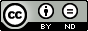Zitieren Sie bitte immer diese URN: urn:nbn:de:kobv:b43-446759

## Application of a Modified Arrhenius Equation to Describe the Time-Temperature Equivalence in Relaxation Analysis of Metal Seals

• For the application of the time-temperature superposition principle a suitable relation is needed to describe the time-temperature shift factor α. Therefore, the Arrhenius equation is widely used due to its simple form and often leads to suitable results. Where, the Arrhenius equation presents a linear relation for the temperature-dependent shift factor in logarithmic scale ln(α) with the absolute inverse temperature (1/ϑ). However, in cases with a large temperature range which eventually include more complex reaction processes, the functional relation between ln(α) and (1/ϑ) is nonlinear in the ‘Arrhenius plot’. In those cases, the monotone change of the nonlinear range in the ‘Arrhenius plot’ can be interpreted as a transient range between two approximately linear or constant regions. An extended application of the modified Arrhenius equation from Nakamura (1989) is presented in this study for this transient range. The introduced method was applied to describe the time-temperatureFor the application of the time-temperature superposition principle a suitable relation is needed to describe the time-temperature shift factor α. Therefore, the Arrhenius equation is widely used due to its simple form and often leads to suitable results. Where, the Arrhenius equation presents a linear relation for the temperature-dependent shift factor in logarithmic scale ln(α) with the absolute inverse temperature (1/ϑ). However, in cases with a large temperature range which eventually include more complex reaction processes, the functional relation between ln(α) and (1/ϑ) is nonlinear in the ‘Arrhenius plot’. In those cases, the monotone change of the nonlinear range in the ‘Arrhenius plot’ can be interpreted as a transient range between two approximately linear or constant regions. An extended application of the modified Arrhenius equation from Nakamura (1989) is presented in this study for this transient range. The introduced method was applied to describe the time-temperature equivalence in the relaxation analysis of restoring seal force of metal seals, which are used in lid-systems of transport and interim storage casks for radioactive materials. But, the method is widely valid and can be used for different objectives which are characterized by thermorheologically simple behavior with nonlinear sensitivity to inverse temperature.Autoren/innen: Linan Qiao, Sven Nagelschmidt, Uwe Herbrich Zeitschriftenartikel Verlagsliteratur Englisch Journal of Civil Engineering and Architecture 2017 3 Gefahrgutumschließungen 3 Gefahrgutumschließungen / 3.4 Sicherheit von Lagerbehältern Bundesanstalt für Materialforschung und -prüfung (BAM) David Publishing Company USA, NY 10989, Valley Cottage 11 9 853 861 Technik, Medizin, angewandte Wissenschaften / Ingenieurwissenschaften / Angewandte Physik Arrhenius equation; Metal seals; Non-linear Arrhenius behavior; Relaxation analysis; Seal force; TTS principle Energie Energie / Kerntechnische Entsorgung https://doi.org/10.17265/1934-7359/2017.09.004 urn:nbn:de:kobv:b43-446759 http://www.davidpublisher.org/index.php/Home/Article/index?id=33931.html 1934-7359 Datei für die Öffentlichkeit verfügbar ("Open Access")Creative Commons - CC BY-ND - Namensnennung - Keine Bearbeitungen 4.0 International 12.04.2018 Nein Wissenschaftliche Artikel der BAM